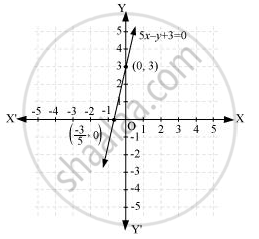Share

# The taxi fare in a city is as follows:- For the first kilometre, the fare is Rs. 8 and for the subsequent distance it is Rs. 5 per km. Taking the distance covered as x km and total fare as Rs y, write a linear equation for this information, and draw its graph. - CBSE Class 9 - Mathematics

ConceptGraph of a Linear Equation in Two Variables

#### Question

The taxi fare in a city is as follows:- For the first kilometre, the fare is Rs. 8 and for the subsequent distance it is Rs. 5 per km. Taking the distance covered as x km and total fare as Rs y, write a linear equation for this information, and draw its graph.

#### Solution

Total distance covered = x km

Fare for 1st kilometre = Rs 8

Fare for the rest of the distance = Rs (x − 1) 5

Total fare = Rs [8 + (− 1) 5]

= 8 + 5x − 5

= 5x + 3

5x − + 3 = 0

It can be observed that point (0, 3) and (-3/5, 0) satisfies the above equation. Therefore, these are the solutions of this equation.

 x 0 -3/5 y 3 0

The graph of this equation is constructed as follows.Here, it can be seen that variable x and y are representing the distance covered and the fare paid for that distance respectively and these quantities may not be negative. Hence, only those values of x and y which are lying in the 1st quadrant will be considered.

Is there an error in this question or solution?

#### APPEARS IN

NCERT Solution for Mathematics Class 9 (2018 to Current)
Chapter 4: Linear Equations in two Variables
Ex. 4.30 | Q: 4 | Page no. 74

#### Video TutorialsVIEW ALL 

Solution The taxi fare in a city is as follows:- For the first kilometre, the fare is Rs. 8 and for the subsequent distance it is Rs. 5 per km. Taking the distance covered as x km and total fare as Rs y, write a linear equation for this information, and draw its graph. Concept: Graph of a Linear Equation in Two Variables.
S